# matplotlib.pyplot.subplot_mosaic#

matplotlib.pyplot.subplot_mosaic(mosaic, *, sharex=False, sharey=False, width_ratios=None, height_ratios=None, empty_sentinel='.', subplot_kw=None, gridspec_kw=None, per_subplot_kw=None, **fig_kw)[source]#

Build a layout of Axes based on ASCII art or nested lists.

This is a helper function to build complex GridSpec layouts visually.

See Complex and semantic figure composition (subplot_mosaic) for an example and full API documentation

Parameters:
mosaiclist of list of {hashable or nested} or str

A visual layout of how you want your Axes to be arranged labeled as strings. For example

```x = [['A panel', 'A panel', 'edge'],
['C panel', '.',       'edge']]
```

produces 4 axes:

• 'A panel' which is 1 row high and spans the first two columns

• 'edge' which is 2 rows high and is on the right edge

• 'C panel' which in 1 row and 1 column wide in the bottom left

• a blank space 1 row and 1 column wide in the bottom center

Any of the entries in the layout can be a list of lists of the same form to create nested layouts.

If input is a str, then it must be of the form

```'''
AAE
C.E
'''
```

where each character is a column and each line is a row. This only allows only single character Axes labels and does not allow nesting but is very terse.

sharex, shareybool, default: False

If True, the x-axis (sharex) or y-axis (sharey) will be shared among all subplots. In that case, tick label visibility and axis units behave as for `subplots`. If False, each subplot's x- or y-axis will be independent.

width_ratiosarray-like of length ncols, optional

Defines the relative widths of the columns. Each column gets a relative width of `width_ratios[i] / sum(width_ratios)`. If not given, all columns will have the same width. Convenience for `gridspec_kw={'width_ratios': [...]}`.

height_ratiosarray-like of length nrows, optional

Defines the relative heights of the rows. Each row gets a relative height of `height_ratios[i] / sum(height_ratios)`. If not given, all rows will have the same height. Convenience for `gridspec_kw={'height_ratios': [...]}`.

empty_sentinelobject, optional

Entry in the layout to mean "leave this space empty". Defaults to `'.'`. Note, if layout is a string, it is processed via `inspect.cleandoc` to remove leading white space, which may interfere with using white-space as the empty sentinel.

subplot_kwdict, optional

Dictionary with keywords passed to the `Figure.add_subplot` call used to create each subplot. These values may be overridden by values in per_subplot_kw.

per_subplot_kwdict, optional

A dictionary mapping the Axes identifiers or tuples of identifiers to a dictionary of keyword arguments to be passed to the `Figure.add_subplot` call used to create each subplot. The values in these dictionaries have precedence over the values in subplot_kw.

If mosaic is a string, and thus all keys are single characters, it is possible to use a single string instead of a tuple as keys; i.e. `"AB"` is equivalent to `("A", "B")`.

New in version 3.7.

gridspec_kwdict, optional

Dictionary with keywords passed to the `GridSpec` constructor used to create the grid the subplots are placed on.

**fig_kw

All additional keyword arguments are passed to the `pyplot.figure` call.

Returns:
fig`Figure`

The new figure

dict[label, Axes]

A dictionary mapping the labels to the Axes objects. The order of the axes is left-to-right and top-to-bottom of their position in the total layout.

## Examples using `matplotlib.pyplot.subplot_mosaic`#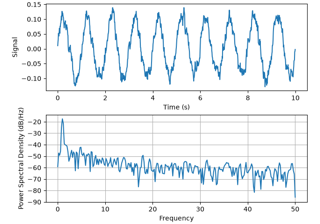Psd Demo

Psd Demo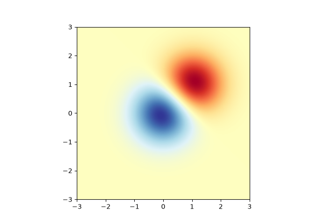Image demo

Image demo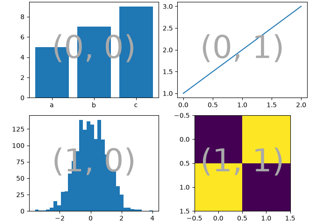Complex and semantic figure composition (subplot_mosaic)

Complex and semantic figure composition (subplot_mosaic)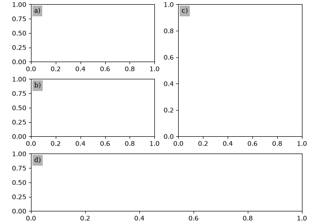Labelling subplots

Labelling subplots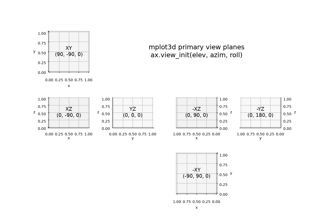Primary 3D view planes

Primary 3D view planes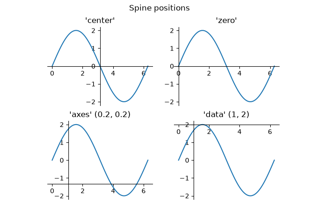Spine placement

Spine placement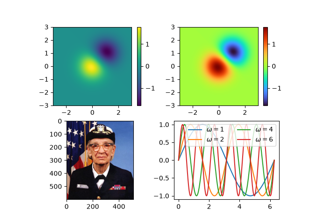mplcvd -- an example of figure hook

mplcvd -- an example of figure hook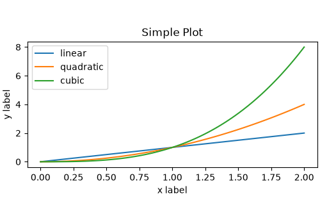Quick start guide

Quick start guide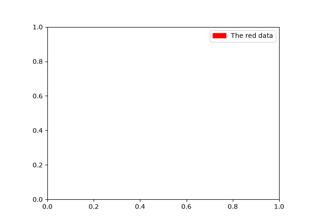Legend guide

Legend guide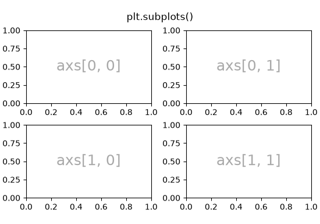Arranging multiple Axes in a Figure

Arranging multiple Axes in a Figure